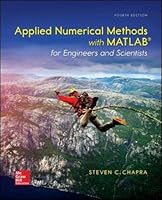# Applied Numerical Methods with MATLAB for Engineers and Scientists, 4th Edition## Book Description

Applied Numerical Methods with MATLAB is written for students who want to learn and apply numerical methods in order to solve problems in engineering and science. As such, the methods are motivated by problems rather than by . That said, sufficient theory is provided so that students come away with insight into the techniques and their shortcomings.

McGraw-Hill Education's Connect, is also available as an optional, add on item. Connect is the only integrated learning system that empowers students by continuously adapting to deliver precisely what they need, when they need it, how they need it, so that class time is more effective. Connect allows the professor to assign homework, quizzes, and tests easily and automatically grades and records the scores of the student's work. Problems are randomized to prevent sharing of answers an may also have a "multi-step solution" which helps move the students' learning along if they experience difficulty.

Part One: Modeling, Computers, and Error

Chapter 1 Modeling, Numerical Methods, and Problem Solving
Chapter 2 MATLAB Fundamentals
Chapter 3 Programming with MATLAB
Chapter 4 Roundoff and Truncation Errors

Part Two: Roots and Optimization
Chapter 5 Roots: Bracketing Methods
Chapter 6 Roots: Open Methods
Chapter 7 Optimization

Part Three: Linear
Chapter 8 Linear Equations and Matrices
Chapter 9 Gauss Elimination
Chapter 10 LU Factorization
Chapter 11 Matrix Inverse and Condition
Chapter 12 Iterative Methods
Chapter 13 Eigenvalues

Part Four: Curve Fitting
Chapter 14 Linear Regression
Chapter 15 General Linear Least-Squares and Nonlinear Regression
Chapter 16 Fourier Analysis
Chapter 17 Polynomial Interpolation
Chapter 18 Splines and Piecewise Interpolation

Part Five: Integration and Differentiation
Chapter 19 Numerical Integration Formulas
Chapter 20 Numerical Integration of
Chapter 21 Numerical Differentiation

Part six: Ordinary Differential Equations
Chapter 22 Initial-Value Problems
Chapter 23 Adaptive Methods and Stiff Systems
Chapter 24 Boundary-Value Problems

Appendix A: Matlab Built-In Functions
Appendix B: Matlab M-File Functions

## Book Details

• Title: Applied Numerical Methods with MATLAB for Engineers and Scientists, 4th Edition
• Author:
• Length: 720 pages
• Edition: 4
• Language: English
• Publisher:
• Publication Date: 2017-02-06
• ISBN-10: 0073397962
• ISBN-13: 9780073397962